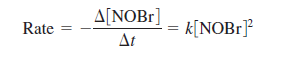# Problem: The rate law for the reaction 2NOBr (g) → 2NO (g) + Br2 (g)at some temperature isa. If the half-life for this reaction is 2.00 s when [NOBr] 0 = 0.900 M, calculate the value of k for this reaction.

###### FREE Expert Solution
92% (130 ratings)
###### Problem Details

The rate law for the reaction

2NOBr (g) → 2NO (g) + Br2 (g)

at some temperature isa. If the half-life for this reaction is 2.00 s when [NOBr] 0 = 0.900 M, calculate the value of k for this reaction.